﻿ 一种基于合作博弈的社区检测算法

# 一种基于合作博弈的社区检测算法A Community Detection Algorithm Based on Cooperative Game

Abstract: With the rapid development of Internet technology, the virtual large-scale social networks are wide- spread. Community structure is an important characteristic of social network, mining the communi-ty structure in large-scale social networks can help us to understand the internal structure and relationships of the network, so as to better apply these networks. Therefore, community detection has important practical significance. A kind of individual rationality as the core of cooperative game community detection algorithm was proposed in this paper, which based on cooperative game com- munity detection model and efficient iterative formula for computing the Shapley value (SH). CDCG algorithm includes initial detection and community adjustment. In the initial detection of changing the strategy environment, every node based on its own maximum SH value to make a decision, after several rounds of decisions, when all of the nodes’ SH value become balance in the network, then the initial detection ended. The characteristics of the internal tight connection of community and rela-tively sparse in communities, by which can detect the unreasonable and meaningless small clusters in step of community adjusting, then the detected cluster has obvious community features. In order to improve efficiency of the algorithm, no contribution nodes pruning and ownership nodes pruning strategies were proposed. Finally, extensive experiments show that CDCG algorithm can automati-cally determine the final number of divided communities, which is effective and efficiency.

1. 引言

2. 相关工作

3. 基于合作博弈的社区检测算法

3.1. 相关定义及定理

$v\left(S\right)=\left\{\begin{array}{l}v\left(S\right)=0\begin{array}{c},\end{array}S\subseteq N,|S|=1,\text{\hspace{0.17em}}\text{or}\text{\hspace{0.17em}}S=\Phi \\ v\left(S\right)=\underset{i\in S}{\sum }\underset{\begin{array}{l}j\in S\\ j\ne i\end{array}}{\sum }\frac{{a}_{ij}}{d\left(i\right)},S\subseteq N,|S|\ge 2,d\left(i\right)\ne 0\end{array}$ (1)

$\begin{array}{l}S{H}_{v}\left(S,i\right)=S{H}_{v}\left({S}_{1},i\right)+\frac{1}{2}\underset{j\in {S}_{2}}{\sum }\left(\frac{{a}_{ij}}{d\left(i\right)}+\frac{{a}_{ji}}{d\left(j\right)}\right),i\in {S}_{1}\\ S{H}_{v}\left(S,j\right)=S{H}_{v}\left({S}_{2},j\right)+\frac{1}{2}\underset{i\in {S}_{1}}{\sum }\left(\frac{{a}_{ij}}{d\left(i\right)}+\frac{{a}_{ji}}{d\left(j\right)}\right),j\in {S}_{2}\end{array}$ (2)

3.2. 初检测算法

1) 初始数据为网络 $G=\left(V,E\right)$ 的拓扑结构，即邻接矩阵 $A$

2) 定义动态集合 ${C}_{result}$，初始化为N个独立节点联盟；初始化一个集合 ${S}_{0}$，其元素为网络中的n个节点，且固定不变。 ${C}_{result}$ 称为当前策略环境。

3) 设置标识布尔型数组Flag[N]，对应每一个节点。标识每一个节点在当前策略环境下合作，获得的SH值是否增大了。

4) 置每个Flag[i] = false，默认值全都为false。

5) 依次循环 ${S}_{0}$ 当中的每一个节点，与当前集合 ${C}_{result}$ 中的不包含自身的其他联盟合作，根据 $S{H}_{v}\left(S,i\right)$ 公式计算获得的SH值，若能获得SH值大于在原来联盟中的SH值，则选择退出原来联盟加入使其获得更大SH值的联盟合作，并设置对应节点Flag[i]为True；若不能，则不做任何操作。

6) 判断 ${S}_{0}$ 中节点是否循环结束，若结束求表达式Flag or Flag or … or Flag[N]的值，如果表达式为True(表示存在任意一个或多个节点的SH值增大了)，跳转执行步骤4、5，否则退出算法，返回初步检测结果 ${C}_{result}$ (表示所有节点的SH值都没有增大，达到SH平衡)。

3.3. 社区调整算法

1) 计算簇 ${C}_{i}$ 的内部边为 ${P}_{in}$，外部边为 ${P}_{out}$

2) 若 ${P}_{out}\ge {P}_{in}$,计算社区 ${C}_{i}$ 与其他各簇之间的连接边数，找出与 ${C}_{i}$ 连接边数最大的簇 ${C}_{j}$

3) 将 ${C}_{i}$${C}_{j}$ 进行合并。

3.4. 基于合作博弈的社区检测算法

$A={\left({a}_{ij}\right)}_{n×n},i,j\in N$，//网络的邻接矩阵

(1) $\text{Flag}\left[n\right]=false$ ;

(2) $S=\left\{\varphi \right\}$ ;//定义一个集合 $S$

(3) $for$ $i=1$ $to$ $n$

(4) ${S}_{i}=\left\{i\right\};S=S\cup \left\{{S}_{i}\right\}$ ;

(5) $endfor$

(6) ${C}_{result}=Initiate\left(A,N\right)$ ;

//初始化联盟，将一个节点作为一个联盟

(7) $While\left(Flag\left[1\right]orFlag\left[2\right]or\cdots orFlag\left[n\right]\right)$

//仍然存在节点在其他联盟中能获得较大值

(7.1) $Flag\left[1\cdots n\right]=false$ ;

(7.2) $For$ $each$ ${S}_{i}\in S$

//测试每一个节点加入各社区,获得最大SH值则加入

(7.2.1) $If$ $Max\left(S{H}_{v}\left({C}_{r}\cup {S}_{i},x\right)\right)>S{H}_{v}\left({C}_{k},x\right)$

$where$ ${C}_{r}\in {C}_{result},x\in {S}_{i}$

(7.2.2) $then$ { ${C}_{r}={C}_{r}\cup {S}_{i}$ ; $Flag\left[i\right]=true$ ;

//如果节点SH值增大，给标识赋值true

$If$ ${S}_{i}\in {C}_{k},then$ ${C}_{k}={C}_{k}-{S}_{i}$ }

//或者直接将该点从 ${C}_{k}$ 中去除。

$endfor$ //end 7.2

$endWhile$ /*end 7

(8) ${C}_{result}=Adjust\left({C}_{result}\right)$ ;//社区调整

(9) $Output:$ ${C}_{result}$ //输出最终社区划分

3.5. 优化剪枝策略

CDCG算法主要时间耗费在初检测过程，但该过程存在冗余计算与比较。因此，为了更好的提高算法的时间效率，根据初检测算法执行策略，定义了无贡献节点和已归属节点。无贡献节点是指一个节点与其合作的对象(节点或簇)无任何连接边。该节点不会对其自身及合作对象产生SH值贡献，可以进行剪枝。已归属节点是指一个节点的度已经全部存在于一个簇中时，它SH值已经不会再增大。因此，该节点已经不需要参加下一轮决策，可以进行剪枝。

3.5.1. 无贡献节点剪枝

$bool$ $Test_Contribution\left(v,C,A\right)\left\{$ /* $v$ 为当前节点， $C$ 为当前联盟， $A$ 为邻接矩阵*/

$Flag=0$ ;

$for$ $i=1$ $to$ $C.Lenght$ //判断是否有边相连

$if$ $A\left[v,C\left[i\right]\right]=1$ $then$

$Flag=1$ ;

$break$ ;

$endif$

$endfor$

$if$ $Flag=1$ { $return$ $true$ ;}

$else$ { $return$ $false$ ;}

$\right\}$

3.5.2. 已归属节点剪枝

$\because$ $d\left(i\right)={d}_{x}\left(i\right)$

$⇒\underset{j=0}{\overset{n}{\sum }}{a}_{ij}={a}_{ik}+{a}_{im}+{a}_{iz}+\cdots$

Þ节点 $i$ 仅与 ${C}_{x}$ 内的节点有连接，则当有任意节点 $p$ 加入当前联盟 ${C}_{x}$ $\left({a}_{ip}={a}_{pi}=0\right)$，根据

$S{H}_{v}\left({C}_{x},i\right)=S{H}_{v}\left({C}_{x},i\right)+\frac{1}{2}\left(\frac{{a}_{ip}}{d\left(i\right)}+\frac{{a}_{pi}}{d\left(p\right)}\right)=S{H}_{v}\left({C}_{x},i\right)$

Þ节点 $i$ 的SH值不再变化且达到最大。

Þ节点 $i$ 为已归属节点。

$bool$ $Test_belong\left(v,C,A\right)\left\{$

// $v$ 为当前节点， $C$ 为联盟， $A$ 为邻接矩阵

${d}_{x}\left(v\right)=0$ ; $d\left(v\right)=\underset{j=1}{\overset{n}{\sum }}A\left[v,j\right]$ ;

$for$ $i=1$ $to$ $C.Lenght$

$if$ $A\left[v,C\left[i\right]\right]=1$

${d}_{x}\left(v\right)++$ ;

$endif$

$endfor$

$if$ ${d}_{x}\left(v\right)=d\left(v\right)$ $then$ { $return$ $true$;}

$else$ { $return$ $false$;}

$\right\}$

4. 实验分析

4.1. 实验数据

1) 128个节点的ad hoc网络。该网络在多篇文章中作为典型基准网络   - ，用于验证社区检测算法的有效性。它主要特点是已知社团结构，具体构造如下：选取n = 128个节点，分成4个社团，每个社团包含32个节点，网络的平均度为16， ${Z}_{out}$ 为某节点与属于其它社团节点之间连接的期望值， ${Z}_{out}$ 越大社区结构越模糊，通过控制参数 ${Z}_{out}$，观察社区划分情况。

$NMI\left(X,Y\right)=\frac{-2{\sum }_{i=1}^{{C}_{X}}{\sum }_{j=1}^{{C}_{Y}}{M}_{ij}\mathrm{log}\left(\frac{n{M}_{ij}}{{M}_{i•}{M}_{•j}}\right)}{{\sum }_{i=1}^{{C}_{X}}{M}_{i}\mathrm{log}\left(\frac{{M}_{i•}}{n}\right)+{\sum }_{j=1}^{{C}_{Y}}{M}_{j}\mathrm{log}\left(\frac{{M}_{•j}}{n}\right)}$

$X$ 为实际的社区划分， $Y$ 为算法得出的社区划分，NMI(X,Y)表示算法得出的社区划分结果与实际社区划分之间的相似程度，取值范围为0~1，其值越大算法所得的社区结构与真实社去结构越接近，NMI = 1表示所得结果与真实社区划分完全一致。NMI=0时表示算法得到的划分与真实划分完全不同。公式中的 $M$ 为一个模糊矩阵，其中行对应真实社区，列对应算法找到的社区。 $M$ 中的一个元素 ${M}_{ij}$ 表示出现在找到的社团 ${C}_{i}$ 中的真实社团 ${C}_{k}$ 中的节点数目。 ${C}_{x}$ 为真实社区的数目， ${C}_{Y}$ 为算法找到的社区的数目。

2) 空手道俱乐部网络。这个网络是由Zachary  在观察一所美国大学空手道俱乐部成员之间的社会联系而构建的。由于俱乐部的管理者和主教练之间发生争吵，于是俱乐部分裂成两个小俱乐部。在空手道俱乐部网络中有34个节点，每个节点表示俱乐部中的一个成员。在文献  中，Zachary给出了该网络自然划分如图3所示，白色和黑色分别代表不同的社区。这个网络广泛地应用于验证网络社区检测的算法。

3) 海豚网络。海豚网络是由Lusseau  对62只尖嘴海豚之间的频繁联系所构成的无向社会网络。这个网络有62个节点，被广泛认同的GN-2社区划分如图8所示。该网络也广泛地应用于验证网络社区检测的算法。

4) LFR基准网络 。LFR基准网络是一种构造的现实主义的网络，能够用于研究社区结构，被广泛应用于测试社区检测算法的性能。LFR基准网络的特点是节点度和社团规模的非均匀性，它能根据用户指定的分布生成更接近真实情况的网络。这里分布不仅包括网络中节点度的分布，而且包括各个社区中节点数的分布。文献  给出了LFR基准网络构造算法，源程序来源于Santo Fortunat的网站： https://sites.google.com/site/santofortunato/inthepress2。

LFR基准网络：LRF(N, k, maxk, mu, minc, maxc)，其中参数N为节点个数，k为平均度，maxk为最大度，mu为节点外部/总度数，minc为最小社区中节点个数，maxc为最大社区中节点个数。调整参数N变化网络的规模，固定其他各参数变量得到不同规模的网络。本文实验中将利用该模型构建不同规模的网络对比算法的时间效率。

4.2. 实验分析

1) 128个节点的ad hoc网络。通过控制Zout，从1变化到8，比较了GN算与本文算法NMI值的变化，如图1所示。由于该网络生成具有随机性，对于每个Zout本文重复做了100次实验，各数值是100次重复试验的平均值。由图可以看出当Zout从1增加8时，该模拟网络社团结构由清晰变得模糊，所以NMI曲线呈现递减趋势。本算法在Zout = 1~4正确划分准确率为100%，Zout ³ 5之后，准确性开始下降，随着Zout值的增大，其下降的速度要快于GN算法。GN算法Zout ³ 6之后，准确性才开始下降。因此本文算法在社区结构比较明显的网络中，检测效果较好。

2) 空手道俱乐部网络。运用该网络比较了本文算法初检测的社区效果与社区调整后的社区效果，比较了GN算法与本文算法的社区检测的结果。从图2图4看出，初检测结果出现了规模小的、不合理的社区，在社区调整后得到了较为合理的社区划分。观察比较图3图4，本文算法在空手道俱乐部网络中划分效果与自然划分有所区别，将{5,6,7,11,17}作为了一个单独的社区划分，节点10划分错误，其他各节点的划分均与实际社区划分一致。与GN算法比较，本文算法划分成了3个社区，GN算法划分成了2个社区，如图5所示。本文算法划分成3个社区，具有合理性。若从模块度( $Q$ 值)的角度网络自然划分时 $Q$ 值为：0.365；GN算法划分最大 $Q$ 值为：0.353；本文算法划分结果的 $Q$ 值为：0.396。从以上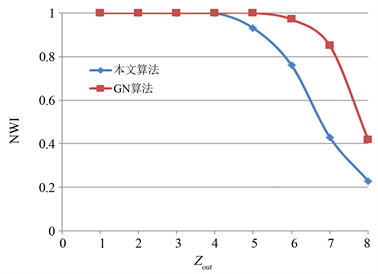Figure 1. Comparison of community detection accuracy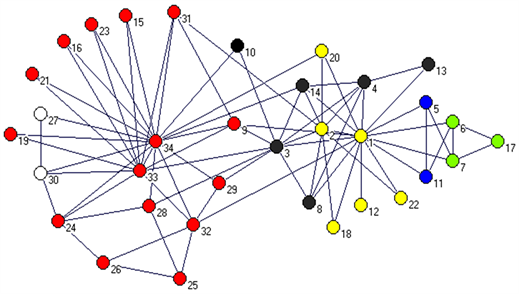Figure 2. Effect of initial detection of the community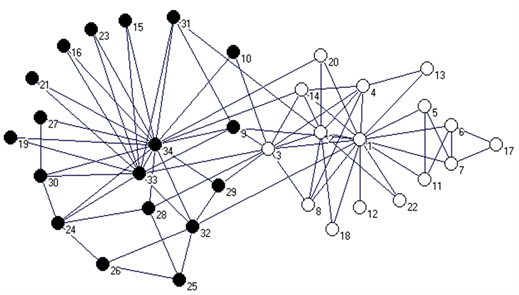Figure 3. Natural division of the Karate Club network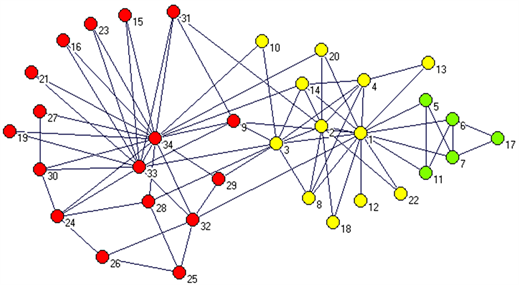Figure 4. The effect of community adjusted community

3) 海豚网络。运用该网络比较了本文算法在初检测的社区效果与社区调整后的社区效果；比较了本文算法与GN算法-2社区状态下的社区检测效果。观察图6图7，可知在初检测结果中由于一些边缘节点及形成闭合回路的节点，造成了一些小的簇出现，在社区调整后，很好的将这些簇进行了归属。从图7图8对比知，仅有节点8，节点20与GN算法-2社区情况下划分不同，说明本文算法在海豚网络中社区划分效果良好。

4) LRF随机基准网络。

$\text{LRF}\left(\text{N},\text{k}=\text{8},\text{maxk}=\text{15},\text{mu}=0.\text{1},\text{minc}=\text{1}0,\text{maxc}=\text{3}0\right);$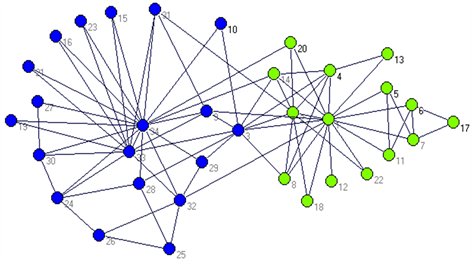Figure 5. GN algorithm-maximum Modularity of community detection effect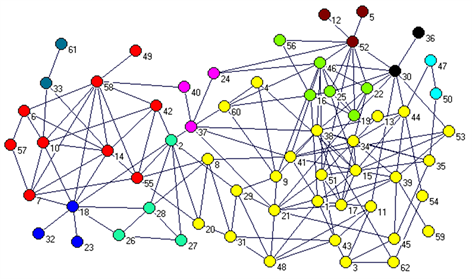Figure 6. Effect of initial detection of the community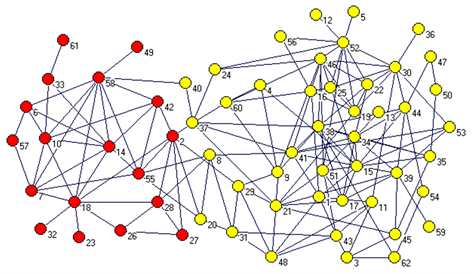Figure 7. The effect of community adjusted community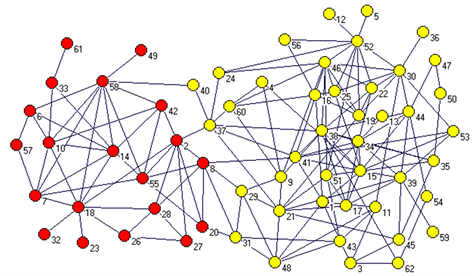Figure 8. GN algorithm-effect of The Dolphin network is divided two community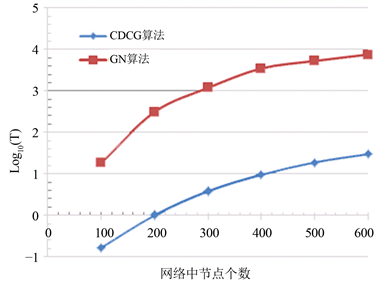Figure 9. Efficiency comparison of GN algorithm and CDCG algorithm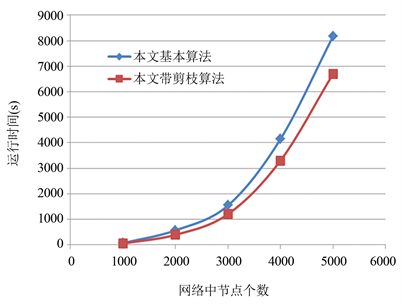Figure 10. Time effect of pruning CDCG algorithm

$\text{LRF}\left(\text{N},\text{k}=\text{15},\text{maxk}=\text{5}0,\text{mu}=0.\text{1},\text{minc}=\text{2}0,\text{maxc}=\text{5}0\right);$

5. 总结

 孟小峰, 余力 (2011) 用社会化方法计算社会. 中国计算机学会通讯, 7, 25-30.

 Zhou, L.H., Cheng, C., Lv, K. and Chen, H.M. (2013) Using coalitional games to detect communities in social networks. Web-Age Information Management, Springer, Berlin, 326-331.

 Girvan, M. and Newman, M.E.J. (2002) Community structure in social and biological networks. Proceedings of the National Academy of Sciences, 99, 7821.

 Zhao, Z.Y., Feng, S.Z., Wang, Q., Huang, Z.X., Williams, G.J. and Fan, J.P. (2012) Topic oriented community detection through social objects and link analysis in social networks. Knowledge-Based Systems, 26, 164-173.

 Shi, C., Yan, Z.Y., Cai, Y.N. and Wu, B. (2012) Multi-objective community detection in complex networks. Applied Soft Computing, 850-859.

 Tasgin, M., Herdagdelen, A. and Bingol, H. (2007) Community detection in complex networks using genetic algorithms.

 Pizzuti, C. (2009) A multi-objective genetic algorithm for community detection in networks. The 21st IEEE International Conference on Tools with Artificial Intelligence.

 Ahn, Y.-Y., Bagrow, J.P. and Lehmann, S. (2010) Link communities reveal multiscale complexity in networks. Nature, 466, Article ID: 09182.

 Chen, W., Liu, Z.M., Sun, X.R. and Wang, Y.J. (2011) Community detection in social networks through community formation games. Proceedings of IJCAI, 3, 2576-2581.

 Fortunato, S. (2010) Community detection in graphs. Physics Reports, 486, 75-174.

 Danon, L., Diaz-Guilera, A., Duch, J. and Arenas, A. (2005) Comparing community structure identification. Journal of Statistical Mechanics: Theory and Experiment, Article ID: P09008.

 Newman, M.E.J. and Girvan, M. (2004) Finding and evaluating community structure in networks. Physical Review E, 69, Article ID: 026113.

 Zachary, W.W. (1977) An information flow model for conflict and fission in small groups. Journal of Anthropological Research, 33, 452-473.

 Lusseau, D. and Newman, M.E.J. (2004) Identifying the role that animals play in their social networks. Proceedings of the Royal Society, 271, 477-481.

 Lancichinetti, A., Fortunato, S. and Radicchi, F. (2008) Benchmark graphs for testing community detection algorithms. Physical Review E, 78, Article ID: 046110.

NOTES

*通讯作者。

 孟小峰, 余力 (2011) 用社会化方法计算社会. 中国计算机学会通讯, 7, 25-30.

 Zhou, L.H., Cheng, C., Lv, K. and Chen, H.M. (2013) Using coalitional games to detect communities in social networks. Web-Age Information Man-agement, Springer, Berlin, 326-331.

 Girvan, M. and Newman, M.E.J. (2002) Community structure in social and biological networks. Proceedings of the National Academy of Sciences, 99, 7821.

 Zhao, Z.Y., Feng, S.Z., Wang, Q., Huang, Z.X., Williams, G.J. and Fan, J.P. (2012) Topic oriented community detection through social objects and link analysis in social networks. Knowledge-Based Systems, 26, 164-173.

 Shi, C., Yan, Z.Y., Cai, Y.N. and Wu, B. (2012) Multi-objective community detection in complex networks. Applied Soft Computing, 850-859.

 Tasgin, M., Herdagdelen, A. and Bingol, H. (2007) Community detection in complex networks using genetic algorithms.

 Pizzuti, C. (2009) A multi-objective genetic algorithm for community detection in networks. The 21st IEEE International Conference on Tools with Artificial Intelligence.

 Ahn, Y.-Y., Bagrow, J.P. and Lehmann, S. (2010) Link communities reveal multiscale complexity in networks. Nature, 466, Article ID: 09182.

 Chen, W., Liu, Z.M., Sun, X.R. and Wang, Y.J. (2011) Community detection in social networks through community formation games. Proceedings of IJCAI, 3, 2576-2581.

 Fortunato, S. (2010) Community detection in graphs. Physics Reports, 486, 75-174.

 Danon, L., Diaz-Guilera, A., Duch, J. and Arenas, A. (2005) Comparing community structure identification. Journal of Statistical Mechanics: Theory and Experiment, Article ID: P09008.

 Newman, M.E.J. and Girvan, M. (2004) Finding and evaluating community structure in networks. Physical Review E, 69, Article ID: 026113.

 Zachary, W.W. (1977) An information flow model for conflict and fission in small groups. Journal of Anthropological Research, 33, 452-473.

 Lusseau, D. and Newman, M.E.J. (2004) Identifying the role that animals play in their social networks. Proceedings of the Royal Society, 271, 477-481.

 Lancichinetti, A., Fortunato, S. and Radicchi, F. (2008) Benchmark graphs for testing community detection algorithms. Physical Review E, 78, Article ID: 046110.

Top